Visitors Online: 77 | Friday 18th October 2019

CBSE Guess > Papers > Question Papers > Class XII > 2006 > Mathematics > Compartment Outside Delhi Set - I.

Mathematics — 2006 (Set I — Compartment Outside Delhi)

SECTION - A

Q. 1.Q. 2. Using properties of determinants, prove the following: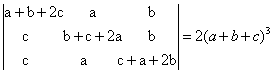Q. 3. In a group of 9 students, there are 5 boys and 4 girls. A team of 4 students is to be selected for a quiz competition. Find the probability that there will be 2 boys and 2 girls in that team.

Q. 4. A and B throw two dice simultaneously turn by turn. A will win if he throws a total of 5, B will win if he throws a doublet. Find the probability that B will win the game, though A started it.

Q. 5.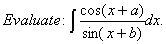Q. 6.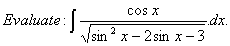Or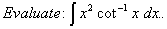Q. 7. The volume of a cube is increasing at the rate of 7 cubic centimeters per second. How fast is the surface area of the cube increasing when the length of an edge is 12 centimeters?

Q. 8.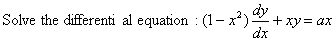Or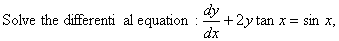.

Q. 9. Examine the validity of the following argument: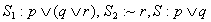Or

Using Boolean algebra simplify the circuit: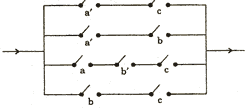Q. 10.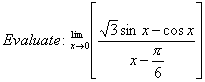Or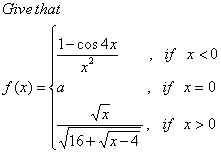If (x) is continuous at x = 0, find the value of a.

Q. 11. DifferentiateQ. 12. Differentiate x sin x w.r.t. x from the first principle.

Q. 13. Find the equations of the tangent and the normal to the curvey y = x3at the point P (1, 1).

Q. 14.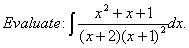Q. 15.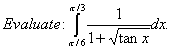Q. 16.find AB and use this result in solving the following system of equations:

Or

Using matrices, solve the following system of linear equations:Q. 17. Prove that the volume of the largest cone that can be inscribed in a sphere of radius R is 8/27 of the volume of the sphere.

Q. 18. Using integration, find the area of the triangle ABC, the coordinates of whose vertices are A (2, 0), B (4, 5) and C (6, 3).

Or

Find the area of the region bounded by the curve y2 = 4x, x = 1, x = 4 and the x-axis in the first quadrant.

SECTION - B

Q. 19. Ifare mutually perpendicular vectors of equal magnitude, show that they are equally inclined to the vector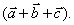Q. 20. Show that the four points A, B, C and D with position vectors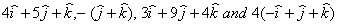respectively are coplanar.

Q. 21. A bullet fires into a target loses half of its velocity after penetrating 3 cm. Find how much further it will penetrate.

Q. 22. The resultant of two velocities u and v is and is v/2 at right angles to u. Prove that the ratio between u and v is,and the angle between a and v is 150°.

Or

A bullet is fired with velocity 98 rn/sec at an angle 30° to the horizontal. Find (i) the time of flight, (ii) the greatest height attained, and (iii) the horizontal range. (Use g = 9.8 rn/sec2)

Q. 23. A plane passes through a fixed point A (a, b, c). Show that the locus of the foot of the perpendicular from the origin on the plane is the sphere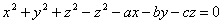.

Q. 24. The resultant of two forces is the same when their directions are inclined at an angle as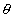when they are inclined at an angle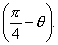Find the value of tan.

Q. 25. In a triangle ABC, three forcesacting along OA, OB, OC respectively are in equilibrium. If O is the circumcentre of the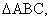prove that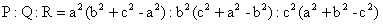Or

The resultant of two like parallel forcesP and Q acting at A and B, 8 cm apart, is 40 N. If the resultant passes through C, where AC = 3 cm, find the magnitude of the forces. Also show that if the forces were interchanged, the resultant shifts through a distance of 2 cm.

Q. 26. 26. A sphere of radius a cm passes through the origin and meets the axes at the points A, B and C. Prove that the locus of the centroid of the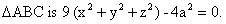SECTION - C

Q. 19. A bill of Rs. 15,000 due on July 13,2005 and another of Rs. 40,000 due on September 24, 2005 are both discounted on May 1,2005. If the difference between the two discounts be Rs. 520, find the rate of discount.

Q. 20. A bill of Rs. 35,000 drawn on April 10, 2005 at 6 months, was discouunted on a certain date at 5% per annum and the proceeds were Rs. 34,300. Find the date on which the bill was discounted.

Q. 21. A car manufacturing factory has two plants X and V. Plant X manufactures 70% of the cars and plant V manufactures 30%. At plant X, 80% of the cars are rated of standard quality and at plant Y, 90% are rated of standard quality. A car is picked up at random and is found to be of standard quality. Find the probability that it has come from plant X.

Q. 22. Find the probability distribution of the number of successes in two tosses of a die, where a success is defined as a number less than 3. Also find the mean and the variance of the distribution.

Or

The probability of hitting a target by A is 1/5. If he fires 5 times, find the probability that he will hit at least two times.

Q. 23. A, B and C enter into partnership in a business with capitals Rs. 5,00,000, Rs. 6,00,000 and Rs. 4,00,000 respectively. A gets 30% of the profit for managing the business and the rest is shared between them in proportion to their capitals. If at the end of the year A gets Rs. 20,000 more than what B and C get together, find the total profit and the shares of B and C in the profit.

Or

A starts a business with a capital of Rs. 6,75,000 and after 6 months B joins investing Rs. 9,75,000; 4 months after that C joins with a capital of Rs. 14,25,000. After a year since the business started the total income was Rs. 15,37,000. They took 10% per annum as interest on the capital they invested and shared the remainder in proportion to their adjusted capitals. Calculate their shares in the profit.

Q. 24. A person has set up a sinking fund in order to have Rs. 3,00,000 after 10 years for his children’s education. How much amount should he set aside half yearly into an account paying interest at the rate of 6% per annum compounded half yearly? [Use (1.03)20 = 1.8030]

Q. 25. x units of a given product can be manufactured at the total cost ofThe selling price per unit isFind the production level at which the profit is maxi mum. Calculate the selling price per unit and the total profit at this level.

Q. 26. A farmer has a supply of chemical fertilizer of type A which contains 10% nitrogen and 6% phosphoric acid and of type B which contains 5% of nitrogen and 10% of phosphoric acid. After soil testing it is found that at least 7 kg of nitrogen and the same quantity of phosphoric acid is required for a good crop. The fertilizer of type A costs Rs. 5.00 per kg and the type B costs Rs. 8.00 per kg. Using linear programming find how many kgs of each type of the fertilizer should be bought to meet the requirement and the cost be minimum. Solve the problem graphically.

 Mathematics 2006 Question Papers Class XII Delhi Outside Delhi Compartment Delhi Compartment Outside Delhi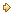Set ISet ISet ISet ISet IISet IISet IISet IISet IIISet III

 CBSE 2006 Question Papers Class XII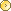EnglishSociologyFunctional EnglishPsychologyMathematicsPhilosopyPhysicsComputer ScienceChemistryEntrepreneurshipBiologyInformatics PracticesGeographyMultimedia & Web TechnologyEconomicsBiotechnologyBusiness StudiesPhysical EducationAccountancyFine ArtsPolitical ScienceHistoryAgriculture